## Example Questions

### Example Question #34 : Circles

A circle of radius, for an angle, has an arc length of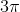. What is the angle?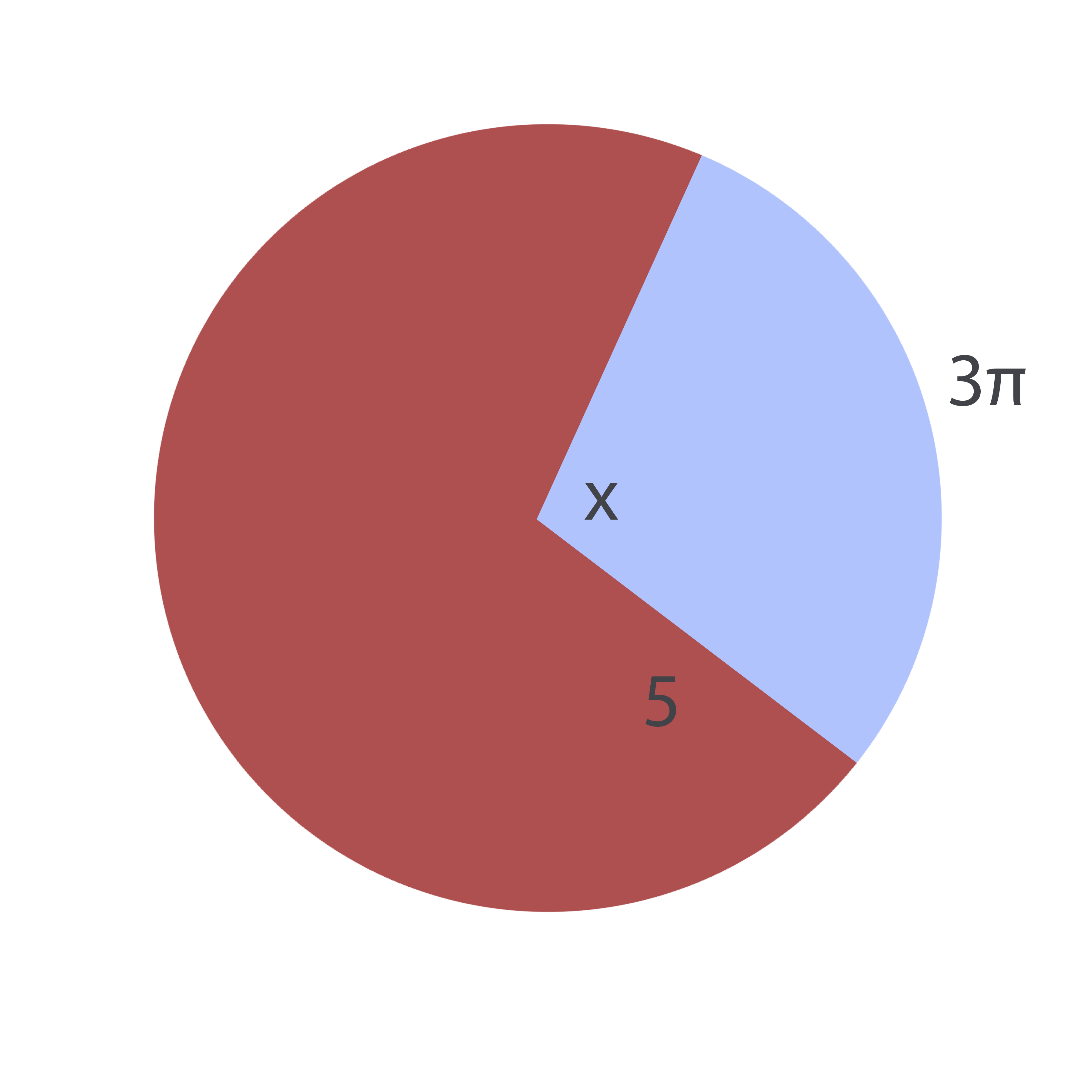Possible Answers: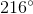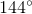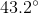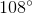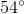Correct answer:Explanation:

The formula for an arc length,, of a circle of a given radius,, and a given angle,is: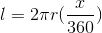Note that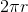is the circumference of the circle.

Conversely, for a known arc length and unknown angle, this equation can be rewritten as follows: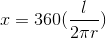Plugging in the given values, it is therefore possible to find the missing angle: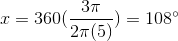### Example Question #35 : Circles

Find the radius of the given circle: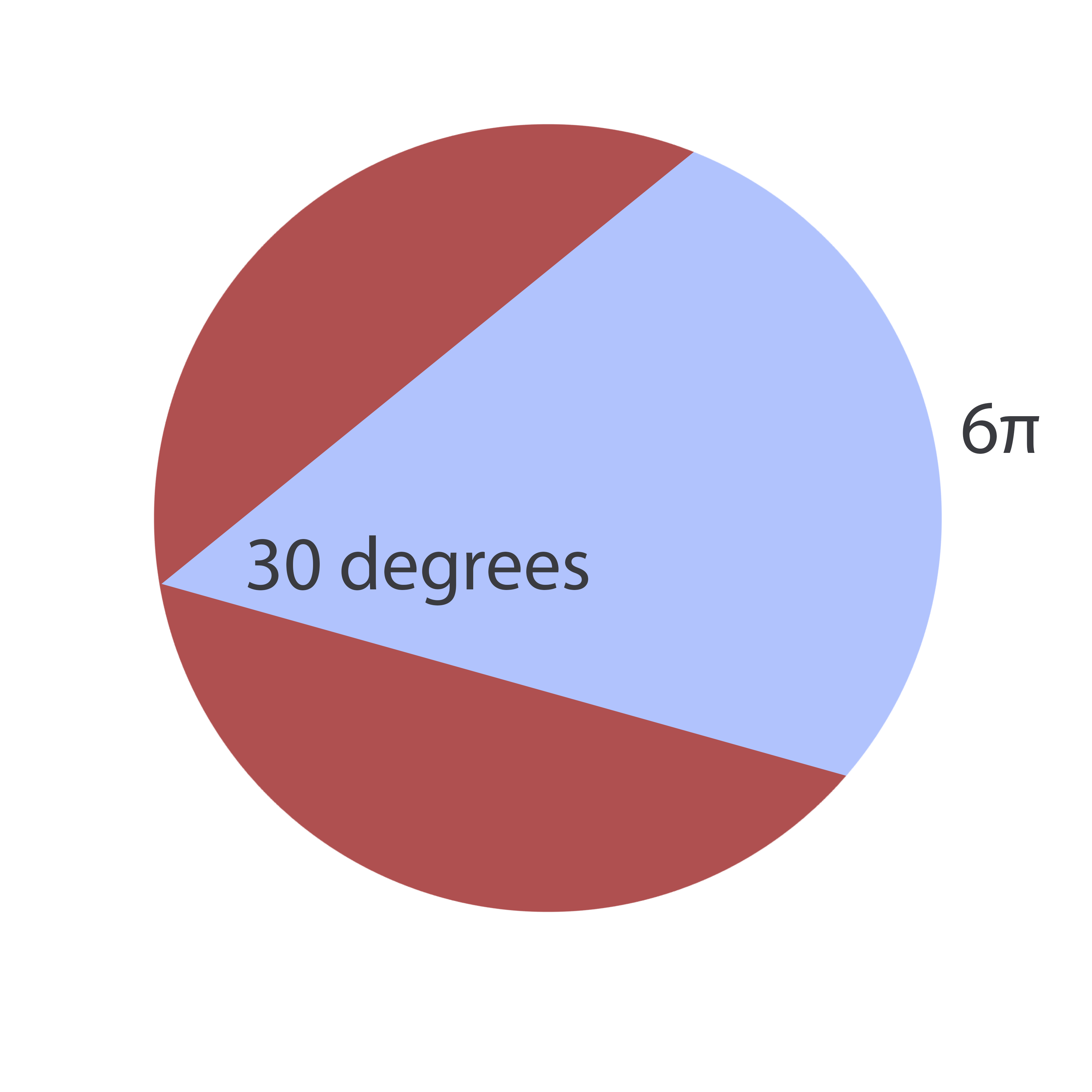Possible Answers:The answer cannot be determined from the information given.Correct answer:Explanation:

To solve this problem, realize that an inscribed angle (an angle formed by two chords) is equal to twice the central angle formed by connecting the origin to the inscribed angle's endpoints: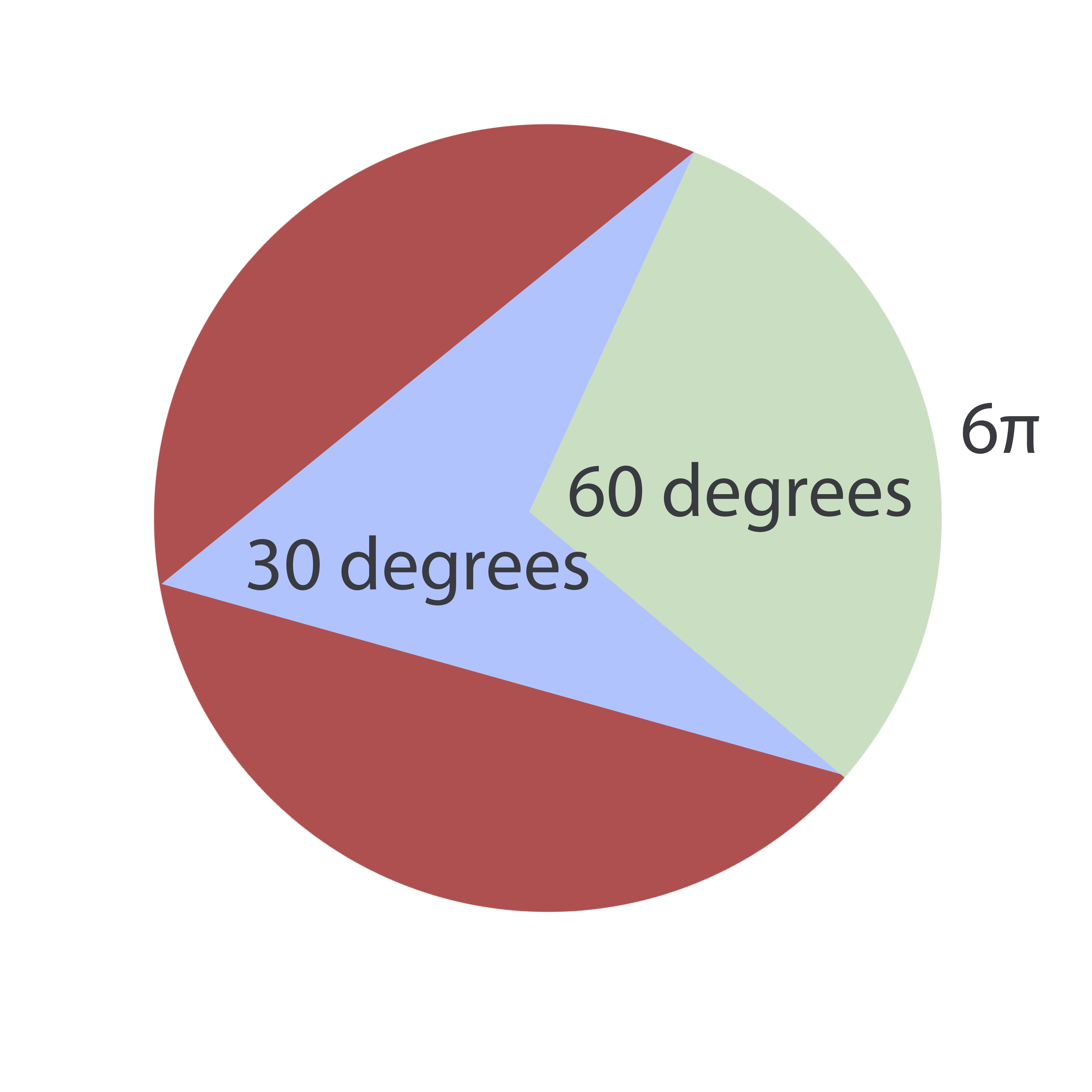Now, the formula for an arc length,, of a circle of a given radius,, and a given angle,is:Since the radius is the unknown, this equation can be rewritten as: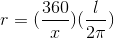Plugging in our values, we find: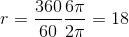### Example Question #36 : Circles

A given pizza with a-inch diameter has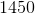calories. A baker cuts the pizza using a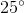angle for each piece. If Susan eats five such pieces, how many calories does she consume? Round to the nearest calorie.

Possible Answers: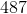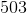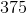Correct answer:Explanation:

To solve this, notice that one piece of pizza comprises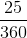of the total pie or (reducing)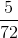of a pie. Now, if Susan buys five slices, she gets: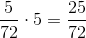of the pie. If the complete pie containscalories, she will then eat the following amount of calories: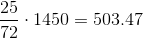Rounding, this iscalories.

### All GRE Math Resources